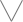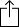C
D
E
F
G
A
B
Chord
Scale
Custom
3nps
CAGED
None
Notes
Degrees
Intervals
None

1
2
3
4
5
6
7
1
2
3
4
5
6
7
8
9
10
11
12
13
14
15
16
17
18
19
20
21
22
23
24
E
B
G
D
A
E
F
G
G
A
A
B
B
C
D
D
E
E
F
G
G
A
A
B
B
C
D
D
E
E
C
D
D
E
E
F
G
G
A
A
B
B
C
D
D
E
E
F
G
G
A
A
B
B
A
A
B
B
C
D
D
E
E
F
G
G
A
A
B
B
C
D
D
E
E
F
G
G
E
E
F
G
G
A
A
B
B
C
D
D
E
E
F
G
G
A
A
B
B
C
D
D
B
B
C
D
D
E
E
F
G
G
A
A
B
B
C
D
D
E
E
F
G
G
A
A
F
G
G
A
A
B
B
C
D
D
E
E
F
G
G
A
A
B
B
C
D
D
E
E

C Harmonic Minor for Guitar - 3nps Chart

C Harmonic Minor, sometimes called "C Aeolian #7" is a diatonic scale and is the 1st mode in the set of Harmonic minor modes

C
D
E
F
G
A
B
CW
H
W
W
H
aug2
H
Play scaleand then